# correlation

(redirected from coefficient number correlation)
Also found in: Dictionary, Thesaurus, Medical, Financial.

## correlation

[‚kär·ə′lā·shən]
(atomic physics)
(geology)
The determination of the equivalence or contemporaneity of geologic events in separated areas.
As a step in seismic study, the selecting of corresponding phases, taken from two or more separated seismometer spreads, of seismic events seemingly developing at the same geologic formation boundary.
(physics)
They tendency of two or more systems that independently exhibit simple behavior to show complex and novel behavior together because of their interaction.
(statistics)
The interdependence or association between two variables that are quantitative or qualitative in nature.
McGraw-Hill Dictionary of Scientific & Technical Terms, 6E, Copyright © 2003 by The McGraw-Hill Companies, Inc.

## correlation

the association between two VARIABLES such that when one changes in magnitude the other one does also, i.e. there is a CONCOMITANT VARIATION. Correlation may be positive or negative. Positive correlation describes the situation in which, if one variable increases, so also does the other. Negative correlation describes the situation in which the variables vary inversely, one increasing when the other decreases.

Correlation can be measured by a statistic, the CORRELATION COEFFICIENT or coefficient of association, of which there exist several forms. Most of these focus on a linear relationship (i.e. a relationship in which the variation in one variable is directly proportional to the variation in the other). When presented graphically, for a perfect relationship between variables a straight line can be drawn through all points on the graph. Correlation coefficients are constructed essentially as measures of departure from this straight line. Curvilinear correlation occurs when the variation of the variables is nonlinear, the rate of change of one being faster than the other.

When no association is found between variables they are said to have statistical independence. The technique of correlation analysis is mainly used on interval level data (see CRITERIA AND LEVELS OF MEASUREMENT), but tests also exist for other levels of data (see SPEARMAN RANK CORRELATION COEFFICIENT).

Finding a correlation does not imply causation. Spurious relationships can be found between variables so there has to be other evidence to support the inference of one variable influencing the other. It also must be remembered that the apparent association may be caused by a third factor influencing both variables systematically For situations in which three or more variables are involved, techniques of MULTIVARIATE ANALYSIS exist. See also REGRESSION, CAUSAL MODELLING, PATH ANALYSIS.

Collins Dictionary of Sociology, 3rd ed. © HarperCollins Publishers 2000
The following article is from The Great Soviet Encyclopedia (1979). It might be outdated or ideologically biased.

## Correlation

in biology, the interdependence of the structure and functions of the cells, tissues, organs, and systems of the body, manifested in the body’s development and in its life activities.

The development and existence of the organism as an integral whole is dependent on correlation. The concept was introduced by G. Cuvier (1800–05); however, since he did not accept the theory of evolution, his idea of correlation had a static character, holding that it was evidence of the permanent coexistence of organs. Evolutionary theory gave correlation a dynamic, historical character: the interconnection of the parts of the body is as much the result of their phylogenic development as of their ontogenic development. The problem of correlation was developed from an evolutionary point of view by A. N. Severtsov, and a more profound understanding of it was offered by I. I. ShmaPgauzen.

Several forms of correlation are distinguished. Genomic correlation is a function of the multiple action of hereditary factors (pleiotropy) and of the action of genes that are more closely interrelated (chromosomal correlation). Morphogenetic correlation is the interdependence among the internal factors of individual development. There may be a connection between two or more morphogenetic processes. Thus, it has been shown that the rudiment of the chordamesoderm becomes the inductor that determines the development of the central nervous system and that the optic cup induces the crystalline lens of the eye. Correlation determines the locus and dimensions of a developing organ. Since morphogenetic processes lead to changes in organic inter-relationships, new morphogenetic correlations develop. Thus, a sequential system of morphogenetic correlations gradually un-folds in the course of individual development, becoming one of the chief factors in ontogeny, maintaining the integrity of the organism throughout its development. The data accumulated by developmental biology have enabled some authors to subdivide these correlations into developmental correlations, which de-pend on the activity of the nervous system; functional (ergontic) correlations; and hormonal correlations. Phylogenetic, or phyletic, correlations—the relational changes of the organs during the course of evolution—were considered by Severtsov to be an independent phenomenon, called coordination.

### REFERENCES

Shmal’gauzen, 1.1. Osnovy sravnitel’noi anatomii pozvonochnykh, 4th ed. Moscow, 1947.
Shmal’gauzen, 1.1. Organizm, kak tseloe v individual’nom i istoricheskom razvitii. Moscow-Leningrad, 1942.
Severtsov, A. N. Morfologicheskie zakonomernosti evoliutsii. Moscow, 1949. (Sobr. soch., vol. 5.)
Balinsky, B. I. An Introduction to Embryology, 2nd ed. Philadelphia-London, 1965.

A. A. MAKHOTIN

## Correlation

in linguistics, the opposition or convergence of linguistic units according to specific features (on all levels of a linguistic system).

Most well developed is the theory of phonological correlation (a phoneme alternation associated with some morphological difference, or forming correlative series that are in opposition according to some one distinctive feature). The notions distinguished include correlative pair (French ã-a, õ-o, ẽ-e, œ̃-œ), feature (nasalization in French, labiovelarization in the Shona languages of the Bantu family), series (ã, õ, ẽ, œ̃), and bundles (in the Archi language, the six-membered bundle z-s-ts-ts’-t̄s-s̄).

## Correlation

in mathematical statistics, a probabilistic or statistical relationship, which, generally speaking, does not have a rigorously functional character. In contrast to a functional relationship, a correlative relationship arises either when one of the random variables depends not only on a given second variable but also on a number of random factors or when, among the conditions upon which one and the other variable depend, there exist some that are common to both of them. A correlation table provides an example of this type of dependence. From Table 1 it is evident that, on the average, an increase in the height of pine trees is accompanied by an increase in the diameter of their trunks; however, pines of a given height (for example, 23 m) possess a distribution of diameters with a fairly large scatter. If, on the average, 23-m pines are thicker than 22-m ones, this relation may be violated to a noticeable extent for individual pines. The statistical correlation in a finite sample being studied is more interesting when it indicates the existence of a link between the phenomena under investigation that conforms to some rule.

Correlation theory is based on the assumption that the phenomena being studied obey some definite probabilistic laws (seePROBABILITY; PROBABILITY THEORY). The relationship between two random events is manifested by the conditional probability of one of the events, given that the other has occurred, being different from the unconditional probability. Similarly, the influence of one random quantity on another is characterized by the laws for the conditional distributions of the first at fixed values of the second. For each possible value X = x, let the conditional expectation y(x) = E(yǀX = x) of the quantity Y be defined. The function y (x) is called the regression of the quantity Y on X, and its graph is called the regression line of Y on X. The dependence of Y on X is manifested in the change in the mean value of Y with a change in X, although for each X = x the quantity Y is still a random quantity with a definite scatter. Let my =E(Y) be the unconditional expectation of Y. If the quantities are independent, then all the conditional expectations of Y are independent of x and coincide with the unconditional expectations:

y(x) = E(YǀX = x) = E(Y) = mY

The converse is not always true. In order to find out how well the regression gives the change in Y with a change in Xt we use the conditional variance of y at a given value of X = x or its mean—the variance of Y relative to the regression line (a measure of the scatter near the regression line):For a strictly functional relationship, the quantity y at a given X = x assumes only one specific value, that is, the variance near the regression line equals zero.

The regression line may be approximately reconstructed from a sufficiently extensive correlation table: one takes for an approximate value of y (x) the mean of those observed values of Y that correspond to the valued = x. Figure 1 depicts the approximate regression line corresponding to data in Table 1 for the dependence of the mean diameter of pine trees on height. In the central part this line is obviously a good expression of the actual dependence. If the number of observations corresponding to certain

Table 1. Correlation between the diameters and heights of 624 northern pine trunks
Height (m)
Diameter (cm)1718192021222324252627282930Total
14–172251          10
18–21133121594       47
22–251113182429147     98
26–29    71830433132   134
30–33    151829351871  114
34–37     13173326126  98
38–41      221019164  53
42–45        41368 132
46–49       337621 22
50–53        14421 12
54–57         111  3
58 and greater          1   1
Total..........4691641578610812491552421624
Mean diameter.....18.518.617.720.022.925.027.230.132.738.340.041.849.543.531.2

values of X is insufficiently large, then this method may lead to completely random results. Thus, the points of the line corresponding to heights of 29 and 30 m are unreliable because of the small number of observations.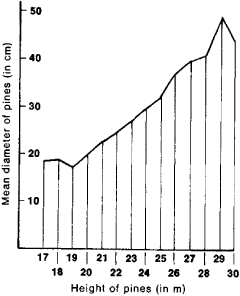Figure 1. Approximate regression line for dependence of mean diameter of northern pine trunks on height

In the case of correlation of two random variables, the usual indicator of the concentration of the distribution near the regression line is the correlation ratio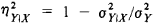where σ2y is the variance of Y (the correlation ratio η2XǀY is analogously defined, but there is no simple relation between ηYǀX and ηXǀY).The quantity η2YǀX, which varies from 0 to 1, is equal to zero if and only if the regression has the form y(x) = my, in which case Y is said to be uncorrelated with X η2Xǀy is equal to unity in the case of an exact functional dependence of Y on X. The most frequently used measure of the degree of dependence between X and Y is the correlation coefficient between X and Y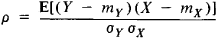where −1 ≤ ρ ≤ 1. However, the practical use of the correlation coefficient as a measure of dependence is justified only when the joint distribution of (X, Y) pairs is normal or approximately normal; the use of p as a measure of dependence between arbitrary Y and X sometimes leads to erroneous deductions, since p can equal zero even when Y depends strictly on X. If the two-dimensional distribution of X and Y is normal, then the regression line of Y on X and that of X on Y are straight lines:

y = my + βy(x − mx) and x = mx + βx(y − my)

where βY = δ(σy/σx) and βx = ρ(σx/σy); βy and βx called the regression coefficients. Moreover,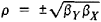Since in this case

E(Y − y(x))2 =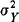(1 − ρ2)

and

E(Y − x(y))2 =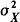(1 − ρ2)

it is evident that p (the correlation ratios coincide with ρ2) completely determines the degree of concentration of the distribution near the regression line: in the limiting case ρ = ±1, the regression lines coalesce into one, which corresponds to the strict linear relationship between Y and X when ρ = 0, the quantities are not correlated.

In the study of the relationship between several random variables X1, . . . , Xn, multiple and partial correlation ratios and correlation coefficients are used (the latter primarily in the case of linear relationships). A fundamental characteristic of the dependence is the set of coefficients ρij —the simple correlation coefficients between Xi and Xj —which form the correlation matrix (ρij) (obviously, ρij = ρij and ρkk = 1). The multiple correlation coefficient serves as a measure of the linear correlation between X and the set of all the remaining variables X2 . . . , Xn for n = 3, it isIf it is assumed that a change in the variables X1 and X2 is determined to some extent by a change in the remaining variables X3, . . . , Xn, then the partial correlation coefficient of X1 and X1 relative to X3, . . . , Xn is an indicator of the linear relationship between X1 and X2 with the effects of X3, . . . , Xn excluded; for n = 3, it is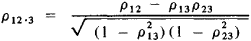Multiple and partial correlation ratios have somewhat more complex expressions.

In mathematical statistics, methods have been developed for estimating the aforementioned coefficients as well as for testing hypotheses concerning their values by using their sample analogs (sample correlation coefficients, correlation ratios). See.

### REFERENCES

Dunin-Barkovskii, I. V., and N. V. Smirnov. Teoriia veroiatnostei i matematicheskaia statistika v tekhnike (the general section). Moscow, 1955.
Cramér, H. Matematicheskie metody statistiki. Moscow, 1948. (Translated from English.)
Hald, A. Matematicheskaia statistika s tekhnicheskimi prilozheniiami. Moscow, 1956. (Translated from English.)
Van der Waerden, B. L. Matematicheskaia statistika. Moscow, 1960. (Translated from German.)
MitropoPskii, A. K. Tekhnika statisticheskikh vychislenii, 2nd ed. Moscow, 1971.

A. V. PROKHOROV

The Great Soviet Encyclopedia, 3rd Edition (1970-1979). © 2010 The Gale Group, Inc. All rights reserved.

## correlation

A confirmation that the target blip seen on radarscope or the track plotted on a plotting board is the same aircraft on which information is being received.